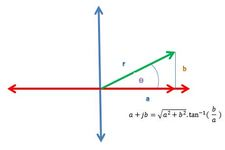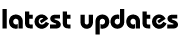Ganit Mantra Series : Complex Number -02

Geometrical Representation of Complex Numbers

A complex number can be represented in a complex plane .Complex plane has two axis ,Real Axis and Imaginary Axis.Argument of a Complex Number

Argument of a non-zero complex number p(z) is defined as an  angle which OP makes with the positive direction of real axis. and is denoted by arg (z)  .

If OP=|z| and arg (z)= θ, then obviously z=r (cos θ + i sin θ), called the polar form of z. ‘Argument of z’ would mean principal argument of z (i.e., argument lying in (-π,π ) unless the context requires otherwise. The general argument of complex number lies in (0,2π ) Thus argument of a complex number z=a+ib = r (cos θ + i sin θ) is the value of θ satisfying

r cos θ = a and r sin θ = b.Let θ = tan-1 |b/a|

Let a point P  be represented by z1=r1 e1,. Where r  is the modulus of the affix P and θ is the argument of the point P….

Complex Numbers -02My Great Web page